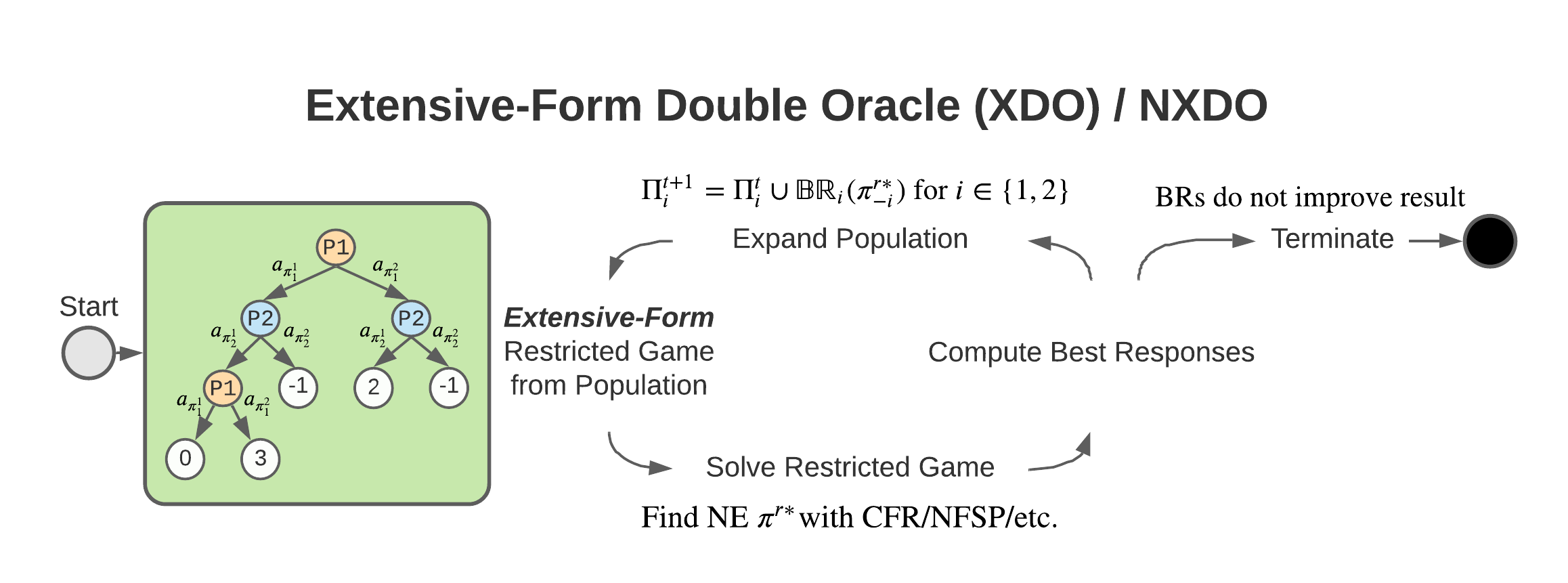## XDO: A Double Oracle Algorithm for Extensive-Form Games

Stephen McAleer, JB Lanier, Kevin Wang, Pierre Baldi, and Roy Fox

35th Conference on Neural Information Processing Systems (NeurIPS), 2021Policy Space Response Oracles (PSRO) is a reinforcement learning (RL) algorithm for two-player zero-sum games that has been empirically shown to find approximate Nash equilibria in large games. Although PSRO is guaranteed to converge to an approximate Nash equilibrium and can handle continuous actions, it may take an exponential number of iterations as the number of information states (infostates) grows. We propose Extensive-Form Double Oracle (XDO), an extensive-form double oracle algorithm for two-player zero-sum games that is guaranteed to converge to an approximate Nash equilibrium linearly in the number of infostates. Unlike PSRO, which mixes best responses at the root of the game, XDO mixes best responses at every infostate. We also introduce Neural XDO (NXDO), where the best response is learned through deep RL. In tabular experiments on Leduc poker, we find that XDO achieves an approximate Nash equilibrium in a number of iterations an order of magnitude smaller than PSRO. Experiments on a modified Leduc poker game and Oshi-Zumo show that tabular XDO achieves a lower exploitability than CFR with the same amount of computation. We also find that NXDO outperforms PSRO and NFSP on a sequential multidimensional continuous-action game. NXDO is the first deep RL method that can find an approximate Nash equilibrium in high-dimensional continuous-action sequential games. Experiment code is available at https://github.com/indylab/nxdo.